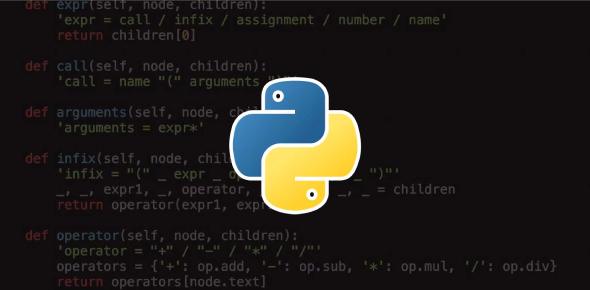# Python Programming Exam Quiz: Trivia!

20 Questions | Attempts: 550Settings.

• 1.
Is Python case sensitive when dealing with identifiers?
• A.

Yes

• B.

No

• C.

Machine dependent

• D.

None of the mentioned

• 2.
Which of the following is invalid?
• A.

_a = 1

• B.

__a = 1

• C.

__str__ = 1

• D.

None of the mentioned

• 3.
Select all options that print hello-how-are-you
• A.

print(‘hello’, ‘how’, ‘are’, ‘you’)

• B.

Print(‘hello’, ‘how’, ‘are’, ‘you’ + ‘-‘ * 4)

• C.

Print(‘hello-‘ + ‘how-are-you’)

• D.

Print(‘hello’ + ‘-‘ + ‘how’ + ‘-‘ + ‘are’ + ‘you’)

• 4.
In order to store values in terms of key and value we use what core datatype.
• A.

List

• B.

Tuple

• C.

Class

• D.

Dictionary

• 5.
Which of the following is incorrect?
• A.

Float(‘inf’)

• B.

Float(‘nan’)

• C.

Float(’56’+’78’)

• D.

Float(’12+34′)

• 6.
What are the values of the following expressions: 2**(3**2) (2**3)**2 2**3**2
• A.

64, 512, 64

• B.

64, 64, 64

• C.

512, 512, 512

• D.

512, 64, 512

• 7.
Carefully observe the code and give the answer.
1. def example(a):
2. a = a + '2'
3. a = a*2
4. return a
5. >>>example("hello")
• A.

Indentation Error

• B.

Cannot perform mathematical operation on strings

• C.

Hello2

• D.

Hello2hello2

• 8.
What is the output of the following? i = 1 while True: if i%3 == 0: break print(i)   i + = 1
• A.

1 2

• B.

1 2 3

• C.

Error

• D.

None of the mentioned

• 9.
What is the output of the following? d = {0: 'a', 1: 'b', 2: 'c'} for x, y in d.items(): print(x, y)
• A.

0 1 2

• B.

A b c

• C.

0 a 1 b 2 c

• D.

None of the mentioned

• 10.
What is the output of the following? for i in range(5): if i == 5: break else: print(i) else: print("Here")
• A.

0 1 2 3 4 Here

• B.

0 1 2 3 4 5 Here

• C.

0 1 2 3 4

• D.

1 2 3 4 5

• 11.
What is the output of the following code ?
1. >>>example = "snow world"
2. >>>print("%s" % example[4:7])
• A.

Wo

• B.

World

• C.

Sn

• D.

Rl

• 12.
Suppose list1 is [1, 3, 2], What is list1 * 2 ?
• A.

[2, 6, 4].

• B.

[1, 3, 2, 1, 3].

• C.

[1, 3, 2, 1, 3, 2] .

• D.

[1, 3, 2, 3, 2, 1].

• 13.
What will be the output?
1. >>>my_tuple = (1, 2, 3, 4)
2. >>>my_tuple.append( (5, 6, 7) )
3. >>>print len(my_tuple)
• A.

1

• B.

2

• C.

5

• D.

Error

• 14.
What will be the output?
1. d = {"john":40, "peter":45}
2. print(list(d.keys()))
• A.

[“john”, “peter”].

• B.

[“john”:40, “peter”:45].

• C.

(“john”, “peter”)

• D.

(“john”:40, “peter”:45)

• 15.
What is the output of the functions shown below? min(max(False,-3,-4), 2,7)
• A.

2

• B.

False

• C.

-3

• D.

-4

• 16.
What is the output of the below program?
1. def sayHello():
2. print('Hello World!')
3. sayHello()
4. sayHello()
• A.

Hello World! Hello World!

• B.

‘Hello World!’ ‘Hello World!

• C.

Hello Hello

• D.

None of the mentioned

• 17.
Is the following code valid? try: # Do something except: # Do something else: # Do something
• A.

No, there is no such thing as else

• B.

No, else cannot be used with except

• C.

No, else must come before except

• D.

Yes

• 18.
What happens if the file is not found in the code shown below? a=False while not a: try: f_n = input("Enter file name") i_f = open(f_n, 'r') except: print("Input file not found")
• A.

No error

• B.

Assertion error

• C.

Input output error

• D.

Name error

• 19.
Which of the following statements are true?
• A.

When you open a file for reading, if the file does not exist, an error occurs

• B.

When you open a file for writing, if the file does not exist, a new file is created

• C.

When you open a file for writing, if the file exists, the existing file is overwritten with the new file

• D.

All of the mentioned

• 20.
The output of the code shown below: import time time.asctime()
• A.

Current date only

• B.

UTC time

• C.

Current date and time

• D.

Current time only

## Related TopicsBack to top
×

Wait!
Here's an interesting quiz for you.